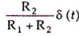# Medium wave radio signals may be received at far off distances at night because

1.  radio waves travel faster at night

2.  ground wave attenuation is low at night

3.  the sky wave is stronger at night

4.  there is no fading at night

4

the sky wave is stronger at night

Explanation :
No Explanation available for this question

# For a short wave radio link between two stations via the ionosphere,the ratio of the maximum usable frequency  to the critical frequency

1.  Is always less than 1

2.  is always greater than1

3.  may be less than or more than 1 depending on the distance between the two stations

4.  does not depend on the distance between the two stations

4

is always greater than1

Explanation :
No Explanation available for this question

# A plane electromagnetic wave travelling along +z-direction, has its electric field given by Ex=2cos(*t) and     Ey=2cos(*t+900).The wave is

1.  Linearly polarized

2.  right circularly polarized

3.  left circularly polarized

4.  lliptically polarized

4

left circularly polarized

Explanation :
No Explanation available for this question

# For a dipole antenna

1.  the radiation intensity is maximum along the normal to the dipole axis

2.  the current distribution along its length is uniform irrespective of the length

3.  the effective length equals its physical length

4.  the input impedance is independent of the location of the feed-point

4

the radiation intensity is maximum along the normal to the dipole axis

Explanation :
No Explanation available for this question

# Relative to a given fixed tree of a network,

1.  Link currents from an independent set

2.  Branch voltage from an independent set

3.  Link currents from an independent set

4.  Branch voltage from an independent set

4

Branch voltage from an independent set

Explanation :
No Explanation available for this question

# For a 2-port network to be reciprocal

1.  Z11=Z22

2.  y21=y12

3.  h21=-h12

4

h21=-h12

Explanation :
No Explanation available for this question

# For the series R-L circuit of the given first figure, the partial fissure diagram at a certain frequency is shown in the given second figure. The operating frequency of the circuit is

1.  Equal to the resonance frequency

2.  Less than the resonance frequency

3.  Greater than resonance frequency

4.  Not zero

4

Not zero

Explanation :
No Explanation available for this question

# For the compensated attenuator of the given figure, the impulse response under the conditionR1 C1 = R2 C2 is

1.

2.

3.

4.

4Explanation :
No Explanation available for this question

# Of the four networks N1,N2,N3 and N4 of the given figure, the networks having identical driving point functions are

1.  N1 and  N1

2.  N2 and N4

3.  N1 and N3.

4.  N1and N4

4

N1 and N3.

Explanation :
No Explanation available for this question

# A linear time invariant system is described by the state variable model

1.  The system is completely controllable

2.  The system is not completely controllable

3.  The system is completely observable

4.  The system is not completely observable

4